EE - 456 / E01 spring 2003

Professor: Arida

Submitted By:

Andrew Buettner

Lab #2: Sampling and Basic Signal Processing

Sunday, April 06, 2003

1) Cover Page 1

3) Objective 3

4) Components Used 3

5) Procedures 3

6) Lab Data / Results 3

1) Diagram 1 4

2) Diagram 2 4

3) Diagram 3 5

4) Diagram 4 5

5) Diagram 5 6

6) Diagram 6 6

7) Diagram 7 7

8) Diagram 8 7

9) Diagram 9 8

10) Diagram 10 8

11) Diagram 11 9

12) Diagram 12 9

13) Diagram 13 10

14) Diagram 14 10

15) Diagram 15 11

16) Diagram 16 11

17) Diagram 17 12

18) Diagram 18 12

19) Diagram 19 13

20) Diagram 20 13

21) Diagram 21 14

22) Diagram 22 14

7) Answers to Lab Questions 15

8) Conclusions 16

9) Attachments 16

• Objective

The objective of this lab is to explore the effects of aliasing and sampling.

• Components Used

1) PC with MatLab® installed

2) Sampling board

3) Spectrum Analyzer #12345

4) Oscilloscope #01-00046

5) Function generator #02-00015

• Procedures

1) Enter command "fir(1)"

2) Enter command "ssrat(10000)"

3) Set the function generator to generate a 1KHz signal with amplitude of 2V.

4) Connect the signal generator to the input of the sampling board.

5) Connect the output of the sampling board to the oscilloscope and the spectrum analyzer.

6) Record the signal spectrum and observe the aliasing that occurs as a result of sampling.

7) Record the resulting signal.

8) Increase the signal frequency to 2KHz.

9) Record the resulting signal and spectrum.

10) Repeat steps 6 and 7 for 4KHz, 5KHz, 6KHz, 8KHz, and 9KHz.

11) Repeat steps 6 and 7 for a 1KHz squarewave and explain the spectrum.

12) Set the function generator to generate a 1KHz sinewave.

13) Enter command "x=ad(100)" to generate a 100 sample array.

14) Plot the resulting function.

15) Rectify the signal using "x1=abs(x);"

16) Plot the new signal.

17) Enter command "da(x1);"

18) Record the resulting waveform and spectrum.

19) Simulate a semi square sawtooth waveform with amplitude 1024 and a frequency of 1KHz.

20) Use the "da(x)" function to realize the waveform.

21) Record the output time signal.

• Lab Data / Results

1) Diagram 1: 1KHz Sinewave Signal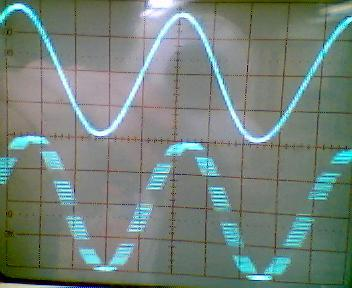2) Diagram 2: 1KHz Sinewave Spectra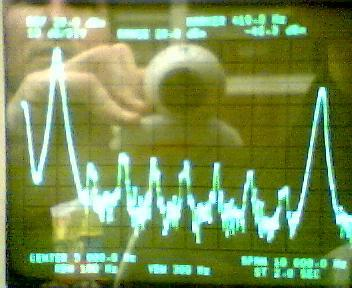3) Diagram 3: 2KHz Sinewave Signal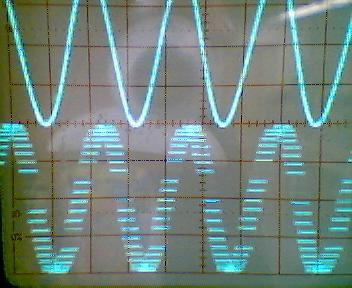4) Diagram 4: 2KHz Sinewave Spectra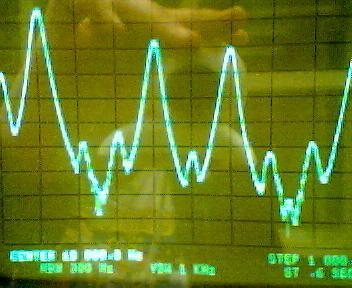5) Diagram 5: 4KHz Sinewave Signal6) Diagram 6: 4KHz Sinewave Spectra7) Diagram 7: 5KHz Sinewave Signal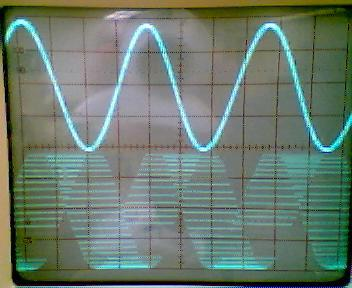8) Diagram 8: 5KHz Sinewave Spectra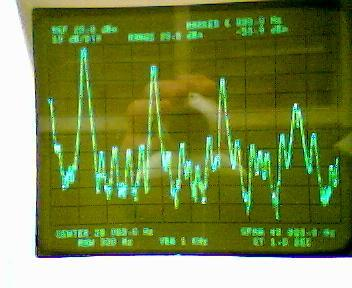9) Diagram 9: 6KHz Sinewave Signal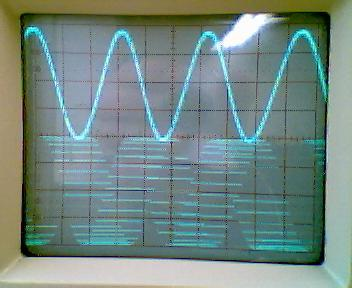10) Diagram 10: 6KHz Sinewave Spectra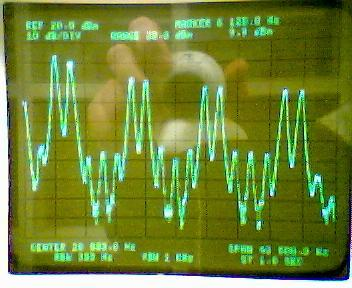11) Diagram 11: 8KHz Sinewave Signal12) Diagram 12: 8KHz Sinewave Spectra13) Diagram 13: 9KHz Sinewave Signal14) Diagram 14: 9KHz Sinewave Spectra15) Diagram 15: 1KHz Square wave Signal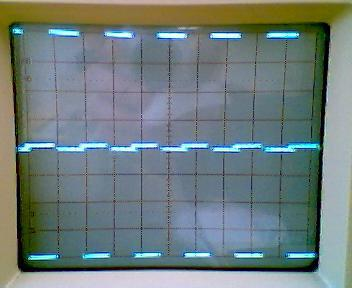16) Diagram 16: 1KHz Square wave Spectra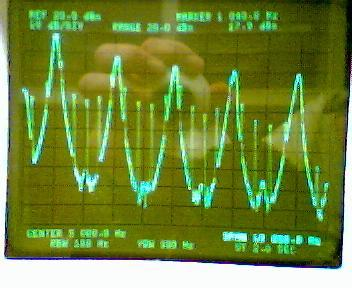17) Diagram 17: Captured Sinewave18) Diagram 18: "Rectified" Signal Output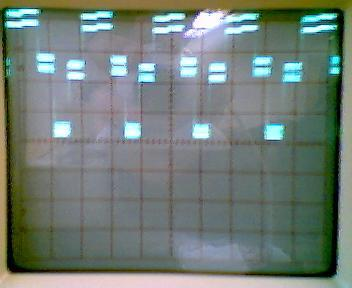19) Diagram 19: "Rectified Signal" before replication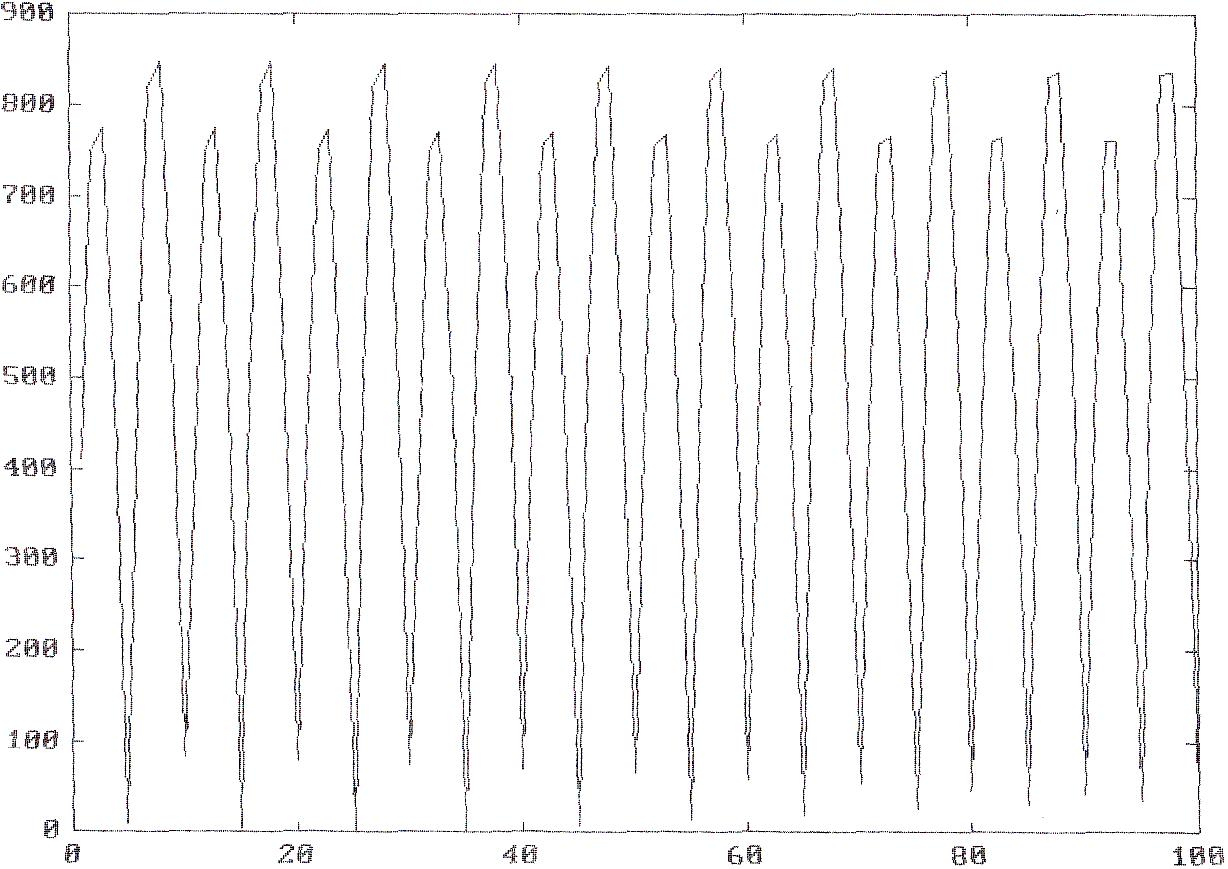20) Diagram 20: "Rectified" signal spectra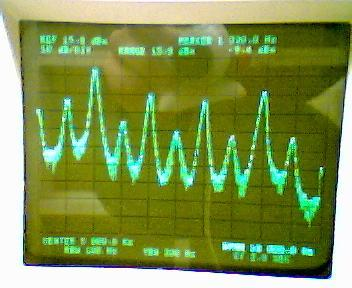21) Diagram 21: Synthesized "Saw tooth" wave.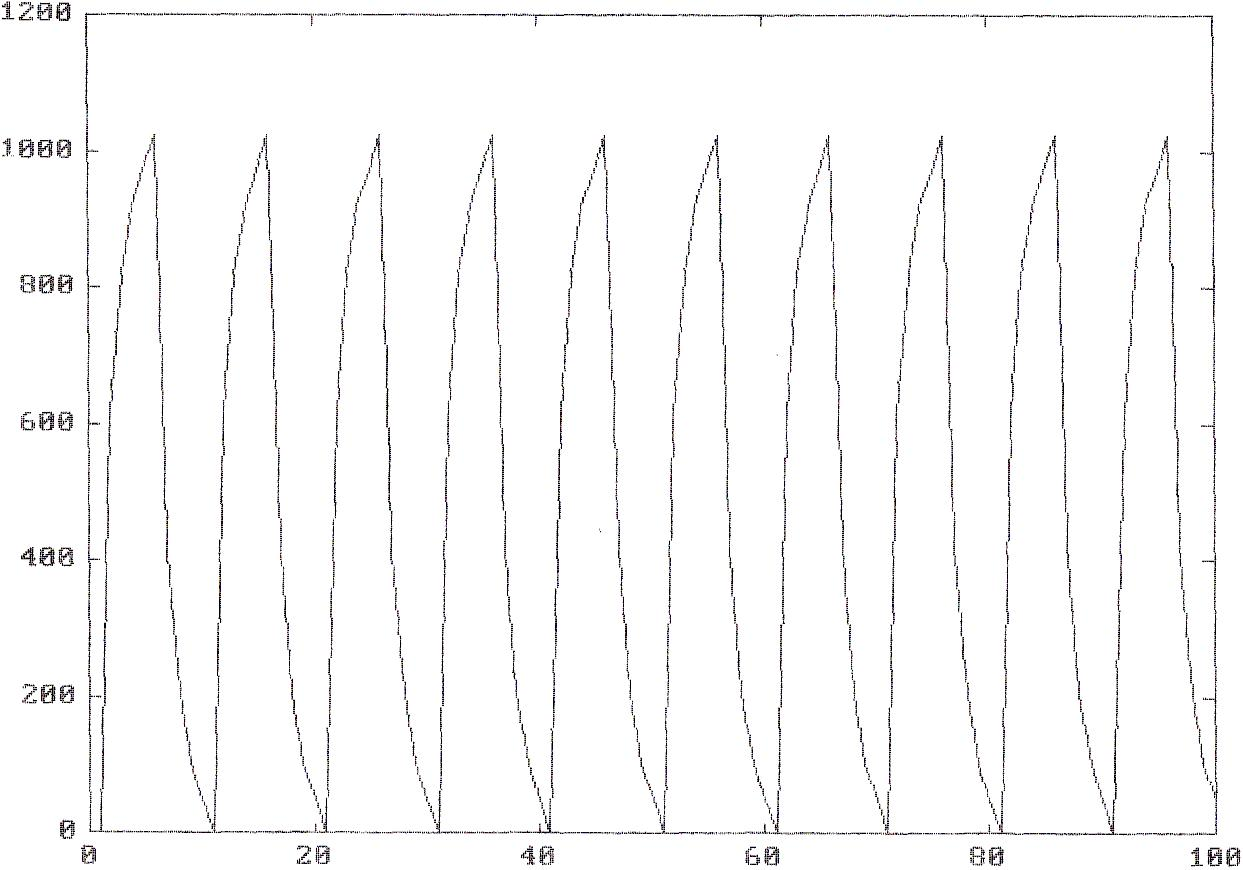22) Diagram 22: Realized "Saw tooth" Wave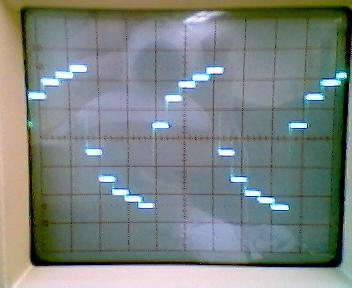1) Q: Describe the spectrum for the following conditions: A signal described by x(t) = 2*cos(wa*t) + cos(wb*t) is sampled at frequency ws. Give the spectrum for the following combinations of wa and wb:

a) wa = .1ws, wb = .2ws

b) wa = .2ws, wb = .4ws

c) wa = .4ws, wb = .6ws

A: a)b)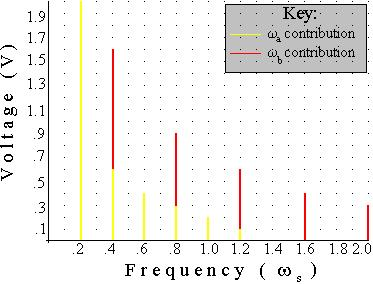c)2) Q: Write MatLab® code to create a square wave off of a sinewave, given array x is a sinewave.

A: x1 = abs(x);

for i = 1 to n, if x(i) = 0

x2(i) = 0;

else

x2(i) = x(i)/x1(i);

• Conclusions

This lab has demonstrated some of the effects that sampling has on a signal. However, some of the effects of signal aliasing were not observed. It is interesting at how much noise is introduced into a signal even though the signal is sampled well below it's Nyquist rate. The answers portrayed in Q1 attempt to show the amount of noise that is introduced. This is expected, and signal generation may also be a source of noise that can not be explained, especially noise that is amplified by the analog to digital conversion, because it is above the Nyquist rate. I did, however, experiment and ran the signal at and above the Nyquist rate. At the Nyquist rate, the signal appeared as a perfect "white" signal, or as straight lines. As the frequency was increased, the noise created shadows, or darker areas that appeared to be a signal at wm - .5ws. This is expected, and is explained within the Nyquist theorem.

• Attachments

1. Original lab handout

2. Original lab data

3. Calculations

4. MatLab® log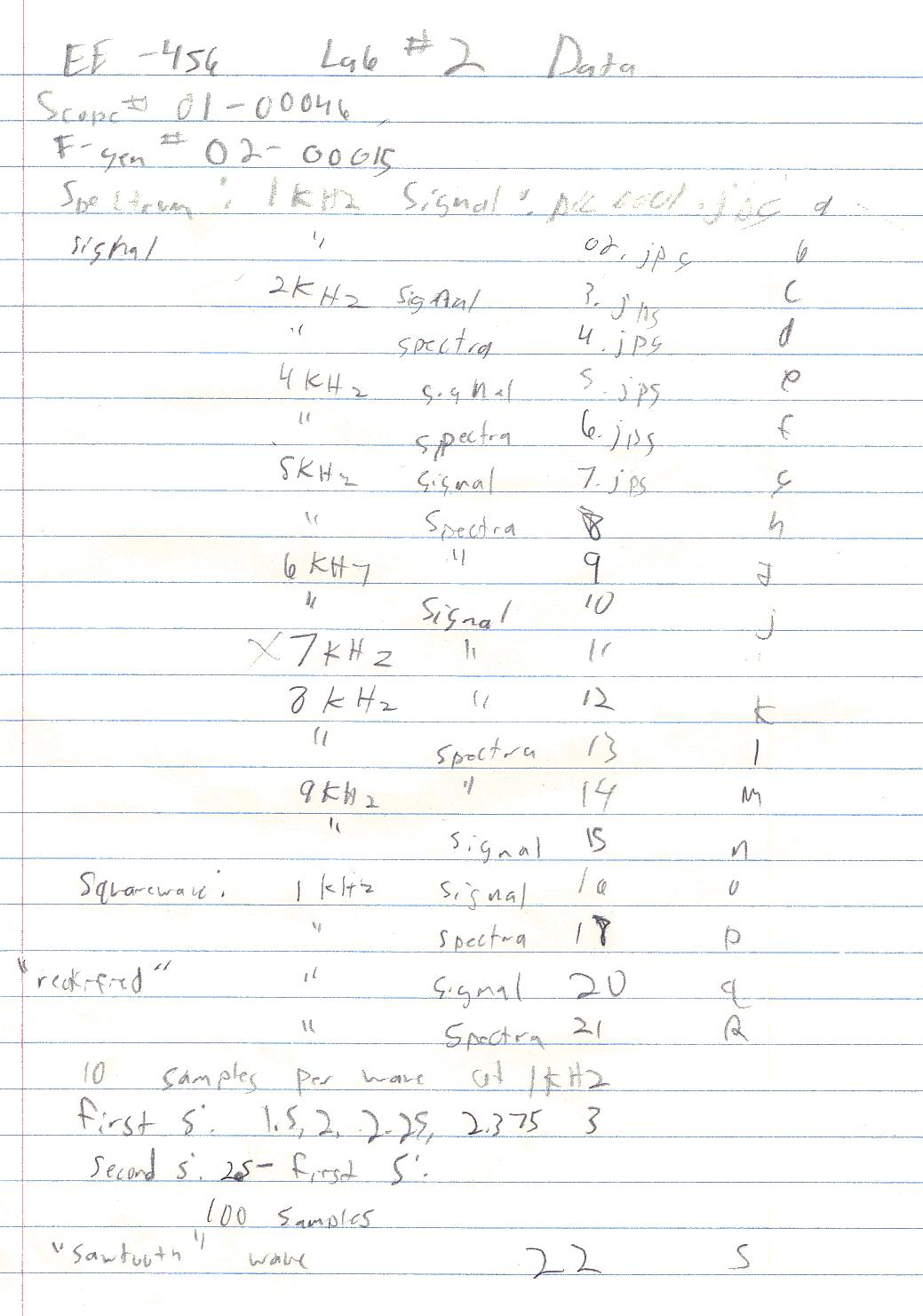fir(1)

¯ ssrat(10000)

¯ plot (x)

¯ x1=abs(x)

x1 =

Columns 1 through 12

410 747 776 491 11 484 818 849 562 84 414 747

Columns 13 through 24

774 487 5 489 820 848 559 79 419 750 773 483

Columns 25 through 36

1 492 821 847 555 75 422 751 771 479 4 497

Columns 37 through 48

824 846 552 69 427 754 771 476 8 500 825 844

Columns 49 through 60

547 64 430 754 768 470 14 506 828 842 544 58

Columns 61 through 72

436 757 768 467 19 509 828 841 538 54 440 757

Columns 73 through 84

765 462 25 515 831 840 535 47 445 760 765 458

Columns 85 through 96

30 518 833 837 530 43 448 761 762 453 35 524

Columns 97 through 100

835 836 527 37

¯ x1=abs(x);

¯ plot(x1)

¯ da(x1)

¯ x2=0:100;

¯ x2(0)=0;

ÛÛÛ Index into matrix is negative or zero.

¯ x2(1)=0;

¯ x2(2)=1.5;

¯ x2(3)=2;

¯ x2(4)=2.25;

¯ x2(5)=2.375;

¯ x(6:10)=2.5-x(1:5)

x =

Columns 1 through 7

-410.0000 -747.0000 -776.0000 -491.0000 -11.0000 412.5000 749.5000

Columns 8 through 14

778.5000 493.5000 13.5000 -414.0000 -747.0000 -774.0000 -487.0000

Columns 15 through 21

-5.0000 489.0000 820.0000 848.0000 559.0000 79.0000 -419.0000

Columns 22 through 28

-750.0000 -773.0000 -483.0000 -1.0000 492.0000 821.0000 847.0000

Columns 29 through 35

555.0000 75.0000 -422.0000 -751.0000 -771.0000 -479.0000 4.0000

Columns 36 through 42

497.0000 824.0000 846.0000 552.0000 69.0000 -427.0000 -754.0000

Columns 43 through 49

-771.0000 -476.0000 8.0000 500.0000 825.0000 844.0000 547.0000

Columns 50 through 56

64.0000 -430.0000 -754.0000 -768.0000 -470.0000 14.0000 506.0000

Columns 57 through 63

828.0000 842.0000 544.0000 58.0000 -436.0000 -757.0000 -768.0000

Columns 64 through 70

-467.0000 19.0000 509.0000 828.0000 841.0000 538.0000 54.0000

Columns 71 through 77

-440.0000 -757.0000 -765.0000 -462.0000 25.0000 515.0000 831.0000

Columns 78 through 84

840.0000 535.0000 47.0000 -445.0000 -760.0000 -765.0000 -458.0000

Columns 85 through 91

30.0000 518.0000 833.0000 837.0000 530.0000 43.0000 -448.0000

Columns 92 through 98

-761.0000 -762.0000 -453.0000 35.0000 524.0000 835.0000 836.0000

Columns 99 through 100

527.0000 37.0000

¯ x(6:10)=2.5-x(1:5);

¯ x2(6:10)=2.5-x2(1:5);

¯ x2(11:20)=x(1:10);

¯ x2(11:20)=x2(1:10);

¯ x2(21:40)=x2(1:20);

¯ x2(41:80)=x2(1:40);

¯ x2(81:100)=x2(1:20);

¯ plot(x2)

¯ x2(100)

ans =

0.1250

¯ x2(101)

ans =

100

¯ x2(101)=0;

¯ plot(x2)

¯ help plot

PLOT Plot vectors or matrices. PLOT(X,Y) plots vector X versus

vector Y. If X or Y is a matrix, then the vector is plotted

versus the rows or columns of the matrix, whichever lines

up. PLOT(X1,Y1,X2,Y2) is another way of producing multiple

lines on the plot. PLOT(X1,Y1,':',X2,Y2,'+') uses a

dotted line for the first curve and the point symbol +

for the second curve. Other line and point types are:

solid - point . red r

dashed -- plus + green g

dotted : star * blue b

dashdot -. circle o white w

x-mark x invisible i

arbitrary c1, c15, etc.

PLOT(Y) plots the columns of Y versus their index. PLOT(Y)

is equivalent to PLOT(real(Y),imag(Y)) if Y is complex.

In all other uses of PLOT, the imaginary part is ignored.

See SEMI, LOGLOG, POLAR, GRID, SHG, CLC, CLG, TITLE, XLABEL

YLABEL, AXIS, HOLD, MESH, CONTOUR, SUBPLOT.

¯

¯ plot(x2)

¯ x3=409.6.*x2;

¯ plot (x3)

¯ plot (x3)

¯ x4(1:100)=x3(1:100);

¯ plot(x4)

¯ da(x4)

¯ quit

117 flop(s).